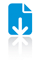# Pre-Reduction Graph Products: Hardnesses of Properly Learning DFAs and Approximating EDP on DAGs

ContentAbstract

The study of graph products is a major research topic and typically concerns the term $f(G*H)$, e.g., to show that $f(G*H)=f(G)f(H)$. In this paper, we study graph products in a non-standard form $f(R[G*H]$ where $R$ is a reduction'', a transformation of any graph into an instance of an intended optimization problem. We resolve some open problems as applications. The first problem is {\em minimum consistent deterministic finite automaton (DFA)}. We show a tight $n^{1-\epsilon}$-approximation hardness, improving the $n^{1/14-\epsilon}$ hardness of [Pitt and Warmuth, STOC~1989 and JACM 1993], where $n$ is the sample size. (In fact, we also give improved hardnesses for the case of {\em acyclic} DFA and NFA.) Due to Board and Pitt [Theoretical Computer Science 1992], this implies the {\em hardness of properly learning DFAs} assuming $NP\neq RP$ (the weakest possible assumption). This affirmatively answers an open problem raised 25 years ago in the paper of Pitt and Warmuth and the survey of Pitt [All 1989]. Prior to our results, this hardness only follows from the stronger hardness of {\em improperly} learning DFAs, which requires stronger assumptions, i.e., either a cryptographic or an average case complexity assumption [Kearns and Valiant STOC~1989 and J.~ACM 1994; Daniely~et~al. STOC 2014]. The second problem is {\em edge-disjoint paths} (EDP) on {\em directed acyclic graphs} (DAGs). This problem admits an $O(\sqrt{n})$-approximation algorithm [Chekuri, Khanna, and Shepherd, Theory of Computing 2006] and a matching $\Omega(\sqrt{n})$ integrality gap, but so far only an $n^{1/26-\epsilon}$ hardness factor is known [Chuzhoy~et~al., STOC~2007]. ($n$ denotes the number of vertices.) Our techniques give a tight $n^{1/2-\epsilon}$ hardness for EDP on DAGs, thus resolving its approximability status. As by-products of our techniques: (i) We give a tight hardness of packing vertex-disjoint $k$-cycles for large $k$, complimenting [Guruswami and Lee, ECCC~2014] and matching [Krivelevich~et~al., SODA~2005 and ACM~Transactions~on~Algorithms~2007]. (ii) We give an alternative (and perhaps simpler) proof for the hardness of properly learning DNF, CNF and intersection of halfspaces [Alekhnovich~et~al., FOCS 2004 and J.~Comput.Syst.~Sci.~2008]. Our new concept reduces the task of proving hardnesses to merely analyzing graph product inequalities, which are often as simple as textbook exercises. This concept was inspired by, and can be viewed as a generalization of, the {\em graph product subadditivity} technique we previously introduced in SODA~2013. This more general concept might be useful in proving other hardness results as well.Top
Authors
• Chalermsook, Parinya
• Laekhanukit, Bundit
• Nanongkai, DanuponTop
Shortfacts
 Category Paper in Conference Proceedings or in Workshop Proceedings Event Title 55th Annual IEEE Symposium on Foundations of Computer Science (FOCS 2014) Divisions Subjects Event Location Philadelphia, USA Event Type Conference Event Dates October 18-21, 2014 Date October 2014 Export BibTeX ASCII Citation DIDL Dublin Core EP3 XML EP3 XML with files embedded EndNote Eprints Application Profile HTML Citation JSON METS MODS Multiline CSV OAI-ORE Resource Map (Atom Format) OAI-ORE Resource Map (RDF Format) OpenURL ContextObject RDF+N-Triples RDF+N3 RDF+XML Refer Reference Manager Simple MetadataTop